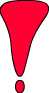COMMON MISCONCEPTIONS

There are some important points that you should focus on carefully. Most of the students have some misconceptions about this subject. Some of them are given below.

• They think that if the velocity is constant than acceleration is also constant. However, as we discussed before, if there is no change in velocity in other word if velocity is constant both in magnitude and direction than the acceleration is zero.
• Another misconception is that if speed of the object is constant than acceleration is zero. As I mentioned before, speed only shows us the magnitude of the velocity. We can not ignore its direction. Thus, acceleration can be zero or not. There is only one option making acceleration zero when speed is constant. If the object does straight line motion with a constant speed then we say acceleration is zero. On the contrary if the object does circular motion with a constant speed we can not talk about zero acceleration. Because, in this situation the direction of the velocity is changing.
• They think that if the object moves with a high speed then its acceleration is also high and if the object moves with a low velocity then the acceleration is also low. But, high velocity does not mean high acceleration. Acceleration can be high, low or zero in high velocities. It is only related to the change in velocity. If the change in velocity high then the acceleration will be high if the change in velocity is low then the acceleration is low.
• Most of the students believe that if the acceleration is positive than the object speeds up, and if the acceleration is negative then the object is slowing down. We can not conclude the motion of the object just looking at its acceleration. We should consider the sign of the velocity while talking about whether it is speeding up or slowing down. If the acceleration is positive and velocity is also positive then we can say that object is speeding up in the positive direction. Moreover, if the acceleration is negative and velocity is also negative we say object is speeding up in negative direction. On the contrary if the velocity and acceleration has opposite directions such as acceleration is positive and velocity is negative or vice versa we say object is slowing down. In summary, to decide if the object is speeding up or slowing down we must look at both directions of the velocity and acceleration.
• Sometimes negative acceleration makes confusion in students’ mind. This negative symbol has physical meaning. We use it to show the direction of the vector quantities.

Kinematics Exams and Solutions

Related